Students get through the MP Board Class 11th Chemistry Important Questions Chapter 1 Some Basic Concepts of Chemistry which are most likely to be asked in the exam.

## MP Board Class 11th Chemistry Important Questions Chapter 1 Some Basic Concepts of Chemistry

Some Basic Concepts of Chemistry Class 11 Important Questions Very Short Answer Type

Question 1.
How are 0.50 mol Na2CO3 and 0.50 M Na2CO3 different?
Solution:
Molar mass of Na2CO3 = (2 × 23) + 12+ (3 × 16)
= 106 g mol-1
Mass of 0.50 mol Na2CO3 = Number of moles × Molar mass
= 0.50 × 106 = 53g Na2CO3
Thus, 0.50 M Na2CO3 means, 53 gm of Na2CO3 is present in 1 litre solution.

Question 2.
What will be the mass of one 12C atom in g?
Solution:
Mass of 1 atom of 12C = $$\frac{\text { Atomic mass of } \mathrm{C}}{\text { Avogadro number }}$$
$$=\frac{12}{6 \cdot 022 \times 10^{23}}$$
= 1.9927 × 10-23g .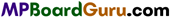Question 3.
What is the law of constant proportion?
The law states that, “A chemical compound is always found to be made up of the same elements combined together in the same proportion by weight.”
Example : Water from different sources is collected and decomposed. After decomposition in each sample of water, it is found that the weight of hydrogen and oxygen is according to the ratio 2 : 16 or 1 : 8.

Question 4.
N2 and H2 combine to form NH3. Under same condition of temperature and pressure ratio in the volume is 1 : 3 : 2. Write the name of the law which proves it.
Or,
Write Gay Lussac’s Law of gaseous volume.
Gay Lussac’s law of combining gaseous volume :
When gases react together, they do so in volumes which bear a simple ratio to one another and to the volumes of the gaseous products provided all measurements were made under similar condition of tem¬perature and pressure.
For example,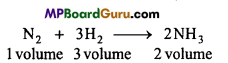The simple ratio in their volume is 1 : 3 : 2.

Question 5.
Write the definition of the following :
(i) Gram atomic mass,
(ii) Gram molecular mass.
(i) Gram atomic mass.:
Gram atomic mass of an element may be defined as the mass, which is numerically equal to its atomic mass, expressed in atomic mass units (a.m.u.).

(ii) Gram molecular mass :
The gram molecular mass of an element is the mass of the substance in gram, which is numerically equal to the molecular mass of substance in atomic mass unit. It is also called one gram molecule of the substance.

Question 6.
What is empirical formula?
Empirical formula is the simple ratio of the atoms of various elements present in one molecule of the compound.
Significance : It represents the ratio between the different atoms of the compound.
Example : Molecular formula of glucose is C6H12O6 ratio between its elements is 1:2:1. Therefore its empirical formula is CH2O.
Molecular formula = (Empirical formula)n
Where, n is an integer i.e.,n= 1,2, 3 etc.

Question 7.
Define Molality of a solution.
Molality of a solution is the number of moles of solute present in 1000 gm of solvent. It is represented by m.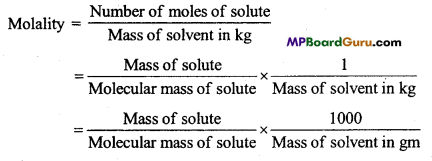Question 8.
What is mole?
The number of elementary particles present in 12 grams of C-12 is called mole. It is equal to 6.023 x 1023 particles. This is known as Avogadro number.
6.023 x 1023 atoms, molecules or ions are called one mole atom, one mole molecule or one mole ions respectively.

Question 9.
What is Limiting Reagent?
The reactant which is present in lesser amount gets consumed first. Such a reactant is called limiting reagent because it limits the product formed.Question 10.
Write the following in scientific notation :
(i) 0.0048
(ii) 234000
(iii) 8008
(iv) 500.0
(v) 6.0012.
(i) 4.8 × 10-3
(ii) 2.34 × 105
(iii) 8.008 × 103
(iv) 5.0 × 102
(v) 6.0012 × 10°

Question 11.
Calculate the molecular mass of the following :
(i) H2O
(ii) CO2
(iii) CH4.
(i) Molecular mass of H2O = 2 × Atomic mass of Hydrogen + 1 × Atomic mass of Oxygen
= 2 × 1 + 1 × 16 = 18

(ii) Molecular mass of CO2 = 1 × Atomic mass of Carbon + 2 × Atomic mass of Oxygen
= 1 × 12 + 2 × 16
= 44

(iii) Molecular mass of CH4 = 1 × Atomic mass of Carbon + 4 × Atomic mass of Hydrogen
= 1 × 12 + 4 × 1
= 16.

Question 12.
What do you understand by Significant figures?
Number of significant figures in a certain value are all the certain digits plus one uncertain digits, For example, number of significant figures in 4.028 are 4.

Question 13.
Tell the number of significant figures in the following :
(i) 0.0025,
(ii) 208,
(iii) 5005,
(iv) 126.000,
(v) 500.00,
(vi) 2.0034.
(i) 2,
(ii) 3,
(iii) 4,
(iv) 3,
(v) 4,
(vi) 5.

Question 14.
How are significant figures rounded up?
For rounding up a number to three significant figures, if fourth number is smaller than 5 then it is left but if the fourth number is 5 or more than 5 then 1 is added to the third significant figure.

Question 15.
Round up the following into three significant figures :
(i) 34.216
(ii) 10.4107
(iii) 0.04597
(iv) 2808.
(i) 34.2
(ii) 10.4
(iii) 0.0460
(iv) 2810.

Question 16.
What are the S.I. Units of mass, Length, Time, Temperature and amount of substance?
Unit of mass is kilogram (kg), length is metre (m), time-second (s), temperature is kelvin (K) and amount of substance is mole (mol).Question 17.
Convert the following into basic units :
(i) 28.7 pm
(ii) 15.15 μs
(iii) 25365 mg.
Solution:
(i) Basic unit of length is metre (m)
2.87 pm × $$\frac{10^{-12} \mathrm{~m}}{1 \mathrm{pm}}$$ = 2.87 × 10-11m

(ii) Basic unit of Time is second (s)
15.15,μs × $$\frac{10^{6} \mathrm{~s}}{1 \mu \mathrm{s}}$$= 1.515 × 10s

(iii) Basic unit of mass is kilogram (kg)
25365 mg × $$\frac{1 \mathrm{~g}}{1000 \mathrm{mg}} \times \frac{\mathrm{lkg}}{1000 \mathrm{~g}}$$ = 2.5365 × 10-2 kg.

Question 18.
If 10 volume of dihydrogen reacts with 5 volume of dioxygen gas then how many volume of water vapour will be obtained?
Solution: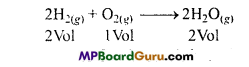According to Gay Lussac’s law of gaseous volume
Volume ratio 2 : 1 : 2
According to the question 10 : 5 : 10
Thus, 10 Volume H2 will react with 5 volume O2 to form 10 volume water vapour.

Question 19.
What is the law of conservation of matter? What is the present position of this theory?
Law of conservation of matter :
In 1744, Lavoisier gave the law of conservation of matter based on the experiments.
This law states that when a chemical reaction takes place, the total mass of the system remains the same before and after the reaction. This law is also referred to as the law of indestructibility of matters, which implies that matter can neither be created nor destroyed by any physical or chemical means.
This law can be proved by experiments.

In the light of modern investigations, law of conservation of matter :
From modern investigations, it is proved that matter can be changed to energy. In some reactions, some energy is produced which causes loss in mass.
According to Einstein’s famous equation of energy-mass relation,
E = mc2
Where, E = energy (in erg), m = mass of the substance (in gm), c = velocity of light (in cm/sec).
In reactions, which we perform in laboratories, very less quantity of energy is absorbed or produced, so the change in mass is very less and is negligible. Such types of changes can be seen clearly in nuclear reaction (atomic bomb). For ordinary reactions, the law is quite valid.

Question 20.
What is the Law of Reciprocal proportion? Explain with example.
Law of reciprocal proportion or Law of Equivalent proportion: This law was put forward by Richter in 1792. It states that:
When two different elements unite with the same quantity of a third element the proportions m which they do so will be the same as, or some simple multiple of the proportions in which they unite with each other.
Or
Weights of two elements (say A and B) which separately combine with a fixed weight of a third element (C) are either the same or simple multiples of the weights (of A and B) in which they combine with each other.

This law can be explained with the help of the following examples:
Consider three elements carbon, oxygen and hydrogen. Element carbon and oxygen combines with a definite mass of hydrogen in the ratio given below :
In methane (CH4), H : C = 4 : 12
and in water (H2O),H : O = 2 : 16 or 4 : 32
According to this law if carbon and oxygen combines with each other then their ratio should be 12 : 32 or some simple multiple of it.
We know that in carbon dioxide, CO2, ratio of C : O = 12 : 32 which is according to this law. Hence, it illustrates law of reciprocal proportion.

Question 21.
In which of the following number of atoms is maximum :
(i) 1gAu(s)
(ii) 1g Na(s)
(iii) 1g Li(s)
(iv) 1g Cl2(g).
Number of moles of substance = $$\frac{\text { Mass of substance }(\mathrm{g})}{\text { Molar mass }}$$
Number of Atoms = Number of moles × Avogadro number
(i) 1gAu(s) = $$=\frac{1}{197}$$ Au mole atoms =$$=\frac{1}{197}$$ × 6.022 × 1023 Au atoms
= 3.057 × 1021 atoms

(ii) 1g Na(s) = $$\frac{6 \cdot 022 \times 10^{23}}{23}$$ = 2.618 × 1022 atoms

(iii) 1g Li(s) = $$\frac{6 \cdot 023 \times 10^{23}}{7}$$ = 8.604 × 1022 atoms

(iv) 1g Cl2(g) = $$\frac{1}{71}$$Cl2 mole molecule = $$\frac{1}{71}$$ × 6.022 × 1023 Cl2 molecule
∴ (1 mole of chlorine contains 2 atoms)

= $$\frac{2}{71}$$ × 6.022 × 1023 Cl atoms
= 1.697 × 1022 atoms
Thus, maximum number of atoms are in 1g Li(s)Question 22.
How is molecular formula of a substance related to its Empirical formula?
Molecular formula is represents the actual number of atoms of various elements present in one mole of a compound.
It is either similar to the empirical formula or a simple multiple of it.
Molecular formula = n (Empirical formula)
n = $$\frac{\text { Molecular mass }}{\text { Empirical formula mass }}$$
Example: The empirical formula of a compound is CH2O and its molecular mass is 180.
n = $$\frac{\text { Molecular mass }}{\text { Empirical formula mass }}$$
$$=\frac{180}{12+12+16}=\frac{180}{30}$$ = 6
Thus, Molecular formula = n x Empirical formula
= 6(CH2O ) = C6H12O6.

Question 23.
(i) What is law of multiple proportion? Explain with an example.
(ii) Why is it necessary to balance chemical equations?
(i) Law of multiple proportion :
When two elements combine to form two or more compounds, different weights of a particular element which combines with definite amount of other element, bear a simple ratio to one another.
Example : Carbon monoxide (CO) and carbon dioxide (CO2) are two compounds of elements carbon and oxygen. The weight of oxygen which combines with a definite weight of carbon (i.e., 12) are 16 and 32 respectively. Thus, the simple ratio of weight of oxygen is 1 : 2, which supports law of multiple proportion.

(ii) It is necessary to balance a chemical equation in order to satisfy the law of conservation of mass according to which the total mass of reactants is equal to the total mass of products.

Question 24.
What is the law of constant composition? How has this law been affected by the discovery of isotopes?
This law was stated by French chemist Louis Proust (1799). It states that ‘A pure chemical compound, regardless of its source, is always made up of the same elements combined together in the fixed proportion by weight.’
Example: Regardless of its source, water is always composed of hydrogen and oxygen combined together in the ratio of 1 : 8 by weight.

Present position of the law :
Discovery of isotopes has proved that an element can have different atoms with different atomic masses but identical properties. Thus there cannot be fixed composition of any compound.
Example : The composition of water is given below :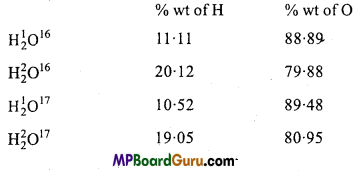These figures are contradictory to the law, however the law is valid as the distribution of isotopes in an element is uniform in the nature.

Question 25.
Use the data given in the following table for calculating the molar mass of Argon available in nature :

 Isotopes Isotopic Molar mass Abundance 36 Ar 35.96775g mol-1 0.337% 36 Ar 37.96272g mol

Solution:
Average molar mass of Ar = Σfi × Ai
= (0.00337 × 35.96755) + (0.00063 × 37.96272) + (0.99600 × 39.9624)
= 0.121 + 0.024 + 39.803
= 39.948 g mol-1.

Question 26.
How many significant figures siinuid he in the following calculations.
(i) $$\frac{0 \cdot 02850 \times 298 \cdot 15 \times 0 \cdot 112}{0 \cdot 5785}$$
(ii) 5 × 5.364
(iii) 0.0125+0.7864+0.0215.

Solution:
(i) In multiplication and di ision in minimum exact number 0.112 is 3 significan
figures. Thus, three (3) significant figures should he in tLe tesult.
$$\frac{0 \cdot 02856 \times 298 \cdot 15 \times 0 \cdot 112}{0 \cdot 5785}$$ = $$\frac{0 \cdot 953698}{0 \cdot 5785}$$
= 1.64857
Three significant figures = 1.65

(ii) In multiplication exact number is 5 and in the second number exact number is four. Thus, there should be 4 significant figures in the result.
5 × 5.364 = 26.82 (4 significant figures)

(iii) In addition and subtraction, in the result should not be more than the number beyond the decimal after addition (or subtraction). Thus, there should be 4 significant figures in the result.
0.0125 + 0.7864 + 0.0215 = 0.8204 Four significant figures.Question 27.
Explain modern concept of Dalton’s atomic theory.
Modem concept of Dalton’s atomic theory are

• According to Dalton, atom is indivisible, but now it is established that atom is divisible. ,
• It is not necessary that weights of atoms of the same element may be same, their weights may be different Amins of the same element havina different weights are called isotopes.
• It is not necessary that atomic massof different elements may be different, e.g., argon and calcium have same atomic mass i,. e., 40 a.m.u. .
• Atoms always do not combine in small numbers and simple ratio. For example, the ratio of C, H and O in sugar (C12H22O11) molecule is 12 : 22 : 11 which is not a simple ratio.
• Atom is the smallest unit which can participate in chemical reaction.
• According to Dalton’s idea, atom is indivisible and it cannot be created: Nowadays breaking of atoms and formation of new atoms is possible by means of nuclear transmutation.

Question 28.
Write the difference between Atom and Molecule.
Differences between Atom and Molecule

 Atom Molecule Molecule 1. Smallest particle of an element, which generally cannot exist in free state. Smallest particle of an element compound which can exist in free state. 2. Participates in chemical reactions. A part of molecule participates in chemical reactions. 3. It cannot be divided in chemical reactions. It can be divided in chemical reactions. 4. All atoms of any element are same. It is formed by combining similar or dissimilar atoms.

Question 29.
Differentiate between Molarity and Molality.
Differences between Molarity and Molality

 Molarity Molality 1. Molarity is related to volume of solution Molality is related to mass of solvent. 2. Molarity depends upon temperature because volume changes with the change in temperature. Molality does not depend on temperature because mass is not affected by temperature. 3. It is denoted by ‘M’ It is denoted by ‘m’

Some Basic Concepts of Chemistry Class 11 Important Questions Long Answer Type

Question 1.
What is chemical equation? Write the ways to balance it with example.
Method of representing a chemical reaction with the help of their chemical formulae and symbols, is called chemical equation.

Balancing of a Chemical Equation :
In general, two methods are used for balancing chemical equations :
1. Hit and trial method :
(i) First of all atoms of that element which covers smallest space are balanced on both sides of the equation.
(ii) Those equations in which oxygen, nitrogen, hydrogen, etc. gases are formed, first of all they are written in atomic state. Such an equation obtained is called atomic equation. Atomic equation is multiplied by 2 to get molecular equation.
Example 1.
Potassium chlorate on heating gives potassium chloride and oxygen.
(a) KClO3 → KCl + O (skeleton equation)

(b) In this equation, right side oxygen atom is multiplied by 3 to get same number of
oxygen atoms on both sides.
KClO3 → KCl + 3O

(c) But in this equation, oxygen is in atomic form. To convert it into molecular form,
the equation is multiplied by 2.
Thus, 2KClO3 → 2KCl + 3O2 (balanced equation)

Example 2.
Copper reacts with sulphuric acid to give copper sulphate, sulphur dioxide
and water.
(a) Cu + H2SO4 → CuS4O + SO2 + H2O (skeleton equation) .

(b) To balance sulphur atoms on both sides, H2SO4 is multiplied by 2.
Cu + 2H2SO4 → CuSO4 + SO2 + H2O

(c) Now to balance hydrogen atoms, H2O is multiplied by 2.
Cu + 2H2SO4 → CuSO4 + SO2 + 2H2O (balanced equation)

2. Partial equation method:
It is generally difficult to balance the complex equations by Hit and Trial method. It is observed that complex equations gets completed in two or more steps. These equations are balanced. If necessary, then partial equation is multiplied
by a whole number. Then, all the partial equations are added in such a way that the intermediate product which is not obtained in the last step gets cancelled. This way, the last equation obtained is correct and balanced.

Example : On heating Cu with cone. H2SO4, copper sulphur, sulphur dioxide and water is formed. This equation can be represented by the following partial equations.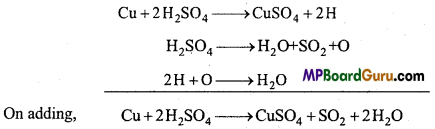Intermediate products 2H and O being present on both the sides of the equations are cancelled.Question 2.
What is the law of multiple proportion? Explain its situation in relation to modern research.
Law of multiple proportion :
This was stated by Dalton in 1803, and was experimentally proved by Berzelius and Stass. It states that, “When two elements combine with each other to form two or more compounds the masses of one of the elements which combine with a fixed mass of the other bear a simple whole number ratio to each other”. For example, Nitrogen forms 5 oxides.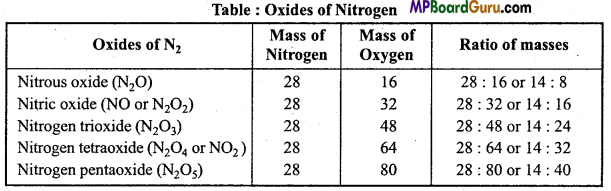Various masses of oxygen 8,16,24,32,40 which combine with a definite mass 14 of nitrogen is in the form of a simple ratio.

Law of multiple proportion in relation to Modem Research:

• Law of multiple proportion can be applicable in that situation when a mass of isotope of an element combines with various masses of a definite isotope of a second element.
• This law is not true for organic compounds.

Question 3.
Explain mole and Avogadro’s number. Write the definition of Atomic mass and molecular mass on the basis of mole concept.
Mole concept:
Atom and molecule are extremely minute particles. In a very small amount of a substance also number of atoms and molecules is very large. For example One milligram (0.001 gram) of carbon contains total 5.019 × 1019 atoms. Generally in chemical studies large amount of substances are used in which number of atoms or molecules is extremely high. It is impossible to count these molecules but its knowledge is essential. Just as 12 pen is a dozen pen, 1000 metre is a kilometre, similarly group of 6.023 × 1023 particles is called one mole. These particles can be molecules atoms or ion.

Thus mole is a unit which represents 6.023 × 1023 particles (molecule, atoms or ion)
Number of molecules in one mole molecule = 6.023 × 1023
Number of atoms in one mole atom = 6.023 × 1023
Number of ions in one mole ion = 6.023 × 1023
It also represents molar mass :
One gram mole oxygen i.e., 32 gram oxygen contain total 6.023 × 1023 molecules. One gram mole sodium i.e., 23 gram sodium contain 6.023 × 1023 atoms.
Formula mass of sodium chloride is 58.5. Thus 58.5 gram sodium chloride contain one mole sodium ion and one mole chloride ion.
“At same temperature and same pressure equal volume of gases contains equal number of atoms.” According to this law, at N.T.P. one gram of all gases contain 6.023 × 1023 atoms. This number is known as Avogadro’s number and it is denoted by N.

Question 4.
What is Stoichiometry? How are the problems based on chemical equations solved by it?
Stoichiometry is derived from the Greek word ‘stoicheion ’ which means element and ‘ metron ’ meaning measure. The word stoichiometry means measurement of amount of element or compound.

In a balanced chemical equation, a quantitative relation exists between the molecules, masses, moles and volume of the reactants and products participating a chemical equation. Problems related to these calculations are of three types :

(a) Involving Mass-Mass relationship :
In such type of problems mass of a product or reactant is given and that of the other is calculated.

(b) Involving Mass-Volume relationship:
In such type of problems mass/volume of a reactant or product is given and that of the other is calculated.

(c) Involving Volume-Volume relationship :
In such type of problems volume of
one reactant or product is given and that of the other is calculated.
To solve the above type of questions (Problems) the following procedure is adopted:

• Balanced chemical equation is written in its molecular form.
• Select the symbols and formulae of those species (atoms or molecules) whose mass/volume are either given or are known by calculation.
• Atomic mass/molecular mass/mole/molar volume of molecules and atoms included in calculation are written.
• By common mathematical calculations amount of the selected substance is calculated.
Think about the following balanced equation :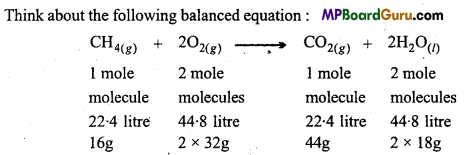Number of molecules (mole) is known as stoichiometric coefficient.Question 5.
Chemical equation :
Method of representing a chemical reaction with the help of chemical formula and symbol of species involved in the reaction called chemical equation. For example, zinc reacts with dilute hydrochloric acid give zinc chloride and hydrogen can
be represented as
Zn + 2HCl → ZnCl2 + H2↑

Limitations Of a chemical equation :
Following informations cannot be obtained from a chemical equation:

• Physical state of reactants and products.
• Concentration of reactants and products cannot be furnished.
• Does not give information about the condition of the reaction,
• No knowledge is obtained about the velocity of the reaction.
• No knowledge about the absorption or evolution of heat of the reaction is obtained.
• Chemical equation does not give any information whether a gas is evolved or a precipitate is formed.
• Chemical equation does not furnish any information about what precautions are necessary for reaction.
• Chemical equation does not furnish any idea about the method of the chemical reaction.
• No information regarding the nature of reaction, i.e., reversible or irreversible.
• It does not provide information about phosphorescence or explosion.
• How much time it will take in completion, this type of information is not obtained.

A chemical equation can be made more clear and informative and useful by balancing
the chemical equations and using the suitable signs. The following are the advantages of it:
(a) Number of reactants and products participating in the reaction.
(b) If atomic mass of the elements are known, then molecular mass of reactants and products can be calculated.
(c) If reactant and products are gaseous, then their volume can be calculated.
(d) It proves the law of conservation of matter.
(e) Valency and Equivalent mass of the elements can be calculated.

Some Basic Concepts of Chemistry Class 11 Important Numerical Questions

Question 1.
Calculate the mass percent of different elements present in sodium sulphate (Na2SO4).
Solution:
Mass percent of Element $$=\frac{\text { Mass of the element in the compound }}{\text { Molar mass of the compound }}$$ × 100
Molar mass of Na2SO4 = (2 × 22.99) + 32.06 + (4 × 16.00)
= 142.04g
Mass percent of Sodium = $$\frac{45 \cdot 98 \times 100}{142 \cdot 04}$$
= 32.37%
Mass percent of Sulphur = $$\frac{32 \cdot 06 \times 100}{142 \cdot 04}$$
= 22.57%
Mass percent of Oxygen $$=\frac{64 \times 100}{142 \cdot 04}$$
= 45.06%.

Question 2.
Determine the Empirical formula of an oxide of iron which has 69.9% iron and 30.1% dioxygen by mass.
Solution: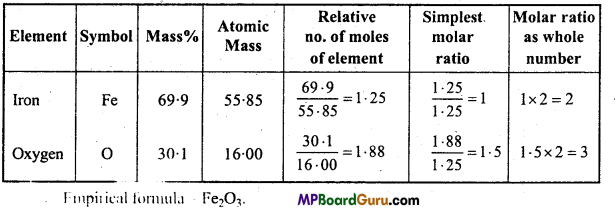Question 3.
Calculate the amount of carbon dioxide that could be produced when :
(i) 1 Mole of carbon is burnt in air.
(ii) 1 mole of carbon is burnt in 16 g of dioxygen.
Solution: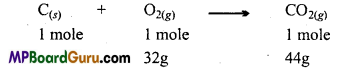(i) According to the above equation on burning 1 mole of carbon completely 44g of CO2 is produced.
(ii) According to the above equation, for burning 1 mole of carbon 32g of oxygen is required. But only 16gm of oxygen is available. Thus, oxygen is the limiting reagent.
Thus, CO2 obtained from 16g of oxygen = $$\frac{44 \times 16}{32}$$
= 22 gm.

Question 4.
Calculate the mass of sodium acetate (CH3COONa) required to make 500 ml of 0.375 molar aqueous solution. Molar mass of sodium acetate is 82.0245 g mol-1.
solution:
Molarity M = $$\frac{w \times 1000}{M \times \text { Volume of solution (in ml) }}$$
Where w = Mass of solute
M = Molar mass of solute
Given
Molarity of solution = 0.375 M
Molar mass of solute = m = 82.0245g mol-1
Volume of solution = 500 ml
Mass of solute w = ?
Mass of solute w = $$\frac{m \times M \times V(\text { in } m l)}{1000}$$
$$\frac{m \times M \times V(\text { in } m l)}{1000}$$
=15.379g
= 15.38g.Question 5.
How much copper can be obtained from 100g of copper sulphate (CuSO4)?
Solution:
Molar mass of CuSO4 = 63.54 + 32.06 + (4 × 16)
= 159.6g mol-1
159.6g of CuSO4 contains Cu = 63.54g
∴ 1 g of CuSO4 will contain Cu = $$\frac{63 \cdot 54}{159 \cdot 6}$$ g
∴ 100g of CuSO4 will contain Cu = $$\frac{63 \cdot 54}{159 \cdot 6}$$ × 100 = 39.81g

Question 6.
Determine the molecular formula of an oxide of iron in which the mass percent of iron and oxygen are 69.9 and 30.1 respectively.
Solution:
First of all Empirical formula is determined,The values given are same.
Empirical formula of iron oxide = Fe2O3
Calculation of Empirical formula mass = (2 × 55.85) + (3 × 16)
= 159.7gmol-1
n = $$$\begin{array}{c} \text { Molar mass } \\ \hline \text { Empirical formula mass } \end{array}$$$
= $$\frac{159 \cdot 7}{159 \cdot 7}$$ = 1
∴ Molecular formula = (Empirical formula),,
= (Fe2O3 )1
= Fe2O3.

Question 7.
Calculate the concentration of nitric acid in moles per litre in a sample which has a density 1.41gm-1 and the mass percent of nitric acid in it being 69%.
Solution:
Molarity = $$\frac{w \times 1000}{\mathrm{M} \times \mathrm{V}_{(\mathrm{ml})}}$$
Given, d = 1.41 gml-1 mass percent of HNO3 = 69%
69% HNO3 means, In 100g solution of HNO3, 69g of HNO3 is present.
Thus, mass of HNO3 (solute) w = 69g
Molar mass of HNO3 = 1.0079 + 14.0067 + (3 × 16.00)
= 63.0146 gmol-1
Density d $$=\frac{m}{V}$$
or
V = $$\frac{m}{d}=\frac{100 \mathrm{~g}}{1 \cdot 41 \mathrm{gml}^{-1}}$$
Molarity = $$\frac{69 \times 1000 \times 1 \cdot 41}{63 \cdot 0146 \times 100}$$ =15.439 M

Question 8.
If the speed of light is 3.0 × 108 ms-1, calculate the distance travelled by light in 2.00ns.
Solution:
2.00ns = 2.00 × 10-9s (1 ns=10-9s)
Distance covered = Velocity × Time
=3.0 × 108ms-1 × 2.0 × 10-9S
= 6.0 × 10-1 = 0.6m.

Question 9.
Calculate the atomic mass (average) of chlorine using the following data :

 % Natural abundance Molar mass 35Cl 75.77 34.9689 37CI 24.23 36.9659

Solution:
Average atomic mass $$\overline{\mathrm{A}}$$, is equal to the product of relative abundance Fi and their corresponding molar mass Ai.
Average atomic mass $$\overline{\mathrm{A}}$$ = Σ fi Ai = F1 × A1 + F2 × A2 + ……………
Thus, average atomic mass of chlorine $$\overline{\mathrm{A}}$$ = 0.7577 × 34.9689 + 0.2423 × 36.9659 g
= 26.4959 + 8.9568
= 35.4527u ≈ 35.5u.

Question 10.
What is the concentration of sugar (C12H22O11) in moT1 if its 20g are dissolved in enough water to make a final volume up to 2L?
Solution:
Molar mass ofsugar(C12H22O11)(M) = (12 x 12) + (22 x 1 .0079) + (11 x 16)
= 342 g mol-1
Given, w = 20g and volume = 2L
Molarity (M) = $$\frac{w}{M \times \text { Volume of solution in litre }}$$
$$=\frac{20}{342 \times 2}$$
= 0.0292 mol L-1Question 11.
If the density of methanol is 0.793 kgL-1, what is the volume needed for making 2.5 L of its 0.25 M solution?
Solution:
Given, d = 0.793 kgL-1 = 0.793 × 103 gL-1
Final volume V2 = 2.5 L
Final Molarity M2 0.25 M
Initial molarity of solution = M1 = ?
Initial volume = V1 = ?
Molar mass of methanol (CH3OH) = (1 × 12) + (4 × 1.0079) + (1 × 16.00)
= 32.0416 ≈ 32gmol-1
Molarity M1 = $$\frac{0.793 \times 10^{3} g \mathrm{~L}^{-1}}{32 \mathrm{~g} \mathrm{~mol}^{-1}}$$ = 24.781 mol L-1
∵ Thus, M1V1 ≡ M2V2
24.781 × V1 = 0.25 × 2.5
V1 = $$\frac{0 \cdot 25 \times 2 \cdot 5}{24 \cdot 781}$$= 0.02522L = 25.22 ml

Question 12.
Pressure is determined as force per unit area of the surface. The S.I. unit of pressure, pascal is shown below :
1 Pa = 1 Nm-2
If mass of air at sea level is 1034 gcm-2. Calculate the pressure in Pascal.
Solution:
Pressure is force or mass per unit area.
Pressure = $$\frac{\text { Force }}{\text { Area }}$$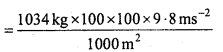= 101332.0 Nm-2[IN = 1kg ms-2]
= 1.01332 × 105 Pa [1Nm-2= 1 pa]

Question 13.
A sample of drinking water was found to be severely contaminated with chloroform CHC13, supposed to be carcinogenic in nature. The level of contamination was 15 ppm (by mass).
(i) Express this in percent by mass.
(ii) Determine the molality of chloroform in the water sample.
Solution:
(i) 15 ppm means :
∵ 106 gram solution contains 15 gm CHCl3
∴ 1 gram solution contains = $$\frac{15}{10^{6}}$$
∴ 100 gram solution contains = $$\frac{15 \times 100}{10^{6}}$$ = 15 x 10-14gm ≈ 1.5 x 10-3%

(ii) Molar mass of CHCl3 = 12 + 1 + (3 × 35.5)
= 119g mol-1
1.5 × 10-3% means 100g of sample contains
1.5 × 10-3g CHCl3
Molarity M = $$\frac{w \times 1000}{m \times \text { Volume of sample }}$$
For water density = $$\frac{1 \cdot 5 \times 10^{-3} \times 1000}{119 \times 100}$$ = 0.000126 ,
= 1.26 × 10-4M.

Question 14.
The following data are obtained when dinitrogen and dioxygen react
together to form different compounds :

 Mass of dinitrogen Mass of dioxygen (i) 14g 16g (ii) 14g 32g (iii) 28g 32g (iv) 28g 80g

(a) Which law of chemical combination is obeyed by the above experimental
data. Give its statement.
(b) Fill in the blanks in the following conversions :
(i) 1km = ………………. mm = …………….. pm
(ii) 1mg = ………………… kg = ……………….. ng
(iii) 1mL = ………………. L = ……………… dm3.
(a) Law of multiple proportion :
According to this law,’ “If two elements combine together to form more than one compound then the masses of one of the element which combine with a fixed mass of the other element is in the form of simple multiple ratio”.
In the given example, in the four oxides if mass of nitrogen is fixed 28g then the ratio of oxygen which combine is 32, 64, 32, 80 which is a simple ratio is 2 : 4 : 2 : 5. Thus, the given data follows the law of multiple proportion.
1km = 1km × $$\frac{1000 \mathrm{~m}}{\mathrm{lkm}}$$ × $$\frac{100 \mathrm{~cm}}{1 \mathrm{~m}}$$ × $$\frac{10 \mathrm{~mm}}{\mathrm{lcm}}$$ 106mm
1km = 1km × $$\frac{1000 \mathrm{~m}}{\mathrm{lkm}}$$ ×$$\frac{1 \mathrm{pm}}{10^{-12} \mathrm{~m}}$$ = 10-15
∴ 1km = 106mm = 1015pm

(ii) 1mg = 1mg × $$\frac{\lg }{1000 \mathrm{mg}}$$ × $$\frac{1 \mathrm{~kg}}{1000 \mathrm{~g}}$$ = 10-610kg
1mg = 1mg × $$\frac{\lg }{1000 \mathrm{mg}}$$ × $$\frac{\ln g}{10^{-9} \mathrm{~g}}$$ = 10610ng
∴ 1mg = 10-610kg = 10610ng

(iii) 1mL = 1mL × $$\frac{1 \mathrm{~L}}{1000 \mathrm{~mL}}$$ = 10-3L
1mL = 1cm3 = 1cm3 × $$\frac{1 \mathrm{dm} \times 1 \mathrm{dm} \times 1 \mathrm{dm}}{10 \mathrm{~cm} \times 10 \mathrm{~cm} \times 10 \mathrm{~cm}}$$ = 103dm3
∴ 1mL = 10-3L = 10-3dm3Question 15.
In a reaction
A + B2 → AB2
Identify the limiting reagent, if any, in the following reaction mixtures :
(1) 300 atoms of A + 200 molecules of B
(ii) 2 mole A +3 mole B
(iii) 100 atoms of A + 100 molecules of B
(iv) 5 mole A + 25 mole B
(v) 2.5 mole A +5 mole B.
Solution:
Limiting reagent first gets consumed (finishes) in a reaction.. Thus, to determine it
amount of A and 13 are compared.
A + B2 → AB2
(i) According to the above equation 1 atom of A reacts with I molecule of B. Thus,
200 atoms of Awil1 react with 200 molecules of B.
Thus, B is the limiting reagert and A will be in excess.

(ii) According to the above equation 1 mole of A reacts with I mole of B. Thus, 2 moles
of A will react with 2 moles of B.
In this condition, A will be the limiting reagent, and B will be in excess.

(iii) According to the above equation I atom of A reacts with 1 molecule of B.
Thus, 100 atoms of A will react with 100 molecules of A.
Thus, it is a stoichiometric mixture.
Thus, there is no limiting reagent neither A nor B.

(iv) In this condition 2.5 mole of A will react with 2.5 mole of B.
Thus, B is limiting reagent and A is in excess.
(v) In this condition A will be the limiting reagent and B will be in excess.

Question 16.
Dinitrogen and dihydrogen react with each other to produce ammonia according to the following chemical equation :
N2(g) + 3H2(g) → 2NH3(g)
(i) Calculate the mass of ammonia produced if 2.00 × 103g dinitrogen reacts with
1.00 × 103g of dihydrogen.
(ii) Will any of the two reactants remain unreacted.
(iii) If yes, which one and what would be its mass.
Solution: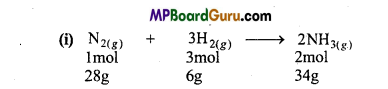∵ 28g of N2 reacts with = 6gH2
∴ 1g N2 will reacts with = $$\frac{6}{28}$$ gH2
∴ 2000g N2 will reacts with = $$\frac{2000 \times 6}{28}$$ = 428.57gH2
Thus, N2 will be the limiting reagent.N2 limits the amount of ammonia produced,
∴ 28g N2 produces = 34g NH3
∴ 1 gN2 produces = $$\frac{34}{28}$$gNH3
∴ 2000 g N2 produces = $$\frac{34}{28}$$ × 2000 = 2428.57g NH3
= 2429g NH3
(ii) H2 will be left. It is in excess.
(iii) Remaining amount of H2= 1000 – 428.57
= 571.43g.

Question 17.
A welding fuel gas contains carbon and hydrogen only. Burning a small sample of it in oxygen gives 3.38g carbon dioxide, 0.690g of water and no other products. A volume of 10.0L (measured at STP) of this welding gas is found to weigh 11.6g. Calculate
(i) Empirical formula,
(ii) Molar mass of the gas and
(iii) Molecular formula.
Solution:
(i) ∵ 44g CO2 = 12g Carbon
∴ 3.38g CO2 = $$\frac{12}{44}$$ × 3.38g = 0.9218g Carbon
∵ 18g H2O = 2g Hydrogen
∴ 0.690g H2O = $$\frac{2}{18}$$ × 0.690g = 0.0767g Hydrogen
Mass of compound = 0.9218 + 0.0767 = 0.9985g
Since, only carbon and hydrogen are present in the compound.
% Amount of C in the compound = $$\frac{0 \cdot 9218}{0 \cdot 9985}$$ × 100 = 92.32%
% Amount of H in the compound = $$\frac{0 \cdot 0767}{0 \cdot 9985}$$ × 100 = 7.68% .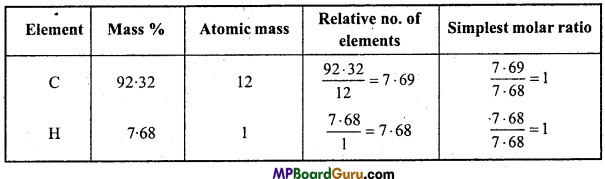Thus, Empirical formula = CH

(ii) Calculation of molecular mass of gas:
∵ At STP, mass of 10.L of gas = 11.6g
∴ At STP, mass of 22.4L of gas =$$\frac{11 \cdot 6 \times 22 \cdot 4}{10 \cdot 0}$$ = 25.984g ≈ 26g mol-1.

(iii) Calculation of Molecular formula:
Empirical formula mass (CH) = 12 + 1 = 13
n = $$\frac{\text { Molecular mass }}{\text { Empirical formula mass }}$$ = $$\frac{26}{13}$$ =2
Molecular formula = (Empirical formula)n
=(CH)2
=C2H2. [n = 2]Question 18.
Calculate the molarity of a solution of ethanol in water in which mole fraction of ethanol is 0.040 (assume the density of water to be 1).
Solution:
Number of moles of solute (Ethanol) in 1 litre solution will be molarity.
1L ethanol solution (dilute solution) = 1L water.
Number of moles of H2O in 1L water = $$\frac{1000 \mathrm{~g}}{18}$$ = 55.55mol
In Binary solution, two components are present.
X1+ X2 = 1
XH2O = 1 – XC2H5OH
XH2O = 1 – 0.040 = 0.96
XH2O = $$\frac{\cdot n_{\mathrm{H}_{2} \mathrm{O}}}{n_{\mathrm{H}_{2} \mathrm{O}}+n_{\mathrm{C}_{2} \mathrm{H}_{5} \mathrm{OH}}}$$
0.96 = $$\frac{55 \cdot 55}{55 \cdot 55+n_{\mathrm{C}_{2} \mathrm{H}_{5} \mathrm{OH}}}$$
On cross multiplication,
(0.96 x 55.55 + 0.96nC2H5OH = 55.55
53.328 + 0.96nC2H5OH = 55.55
0.96nC2H5OH = 55.55 – 53.328 = 2.222
nC2H5OH = $$\frac{2 \cdot 222}{0 \cdot 96}$$
2.3145 mol.

Question 19.
Calcium carbonate reacts with aqueous HCl to give CaCl2 and C02 according to the reaction :
CaCO3(s) +2HCL(aq) → CaCl2(aq) + CO2(g) + H2O(l)
What mass of CaCO3 is required to react completely with 25ml of 0.75 M HCl?
Solution:
(i) Mass of 25 ml of 0.75 M HCl
Molarity = $$\frac{w \times 1000}{m \times V(m l)}$$
0.75 = $$\frac{w \times 1000}{36 \cdot 5 \times 25}$$
Molecular mass of HCl = 1 + 35.5 36.5 g/mol
w = 0.75 × 36.5 $$\frac{25}{1000}$$ = 0.6844g.

(ii) CaCO3(s) + 2HCl(aq) ) → CaCl2(aq) + CO2(g) + H2O(1)
100g 2 × 36.5 = 73g
∵ 73g HC1 completely reacts with = 100g CaCO3
∴ 1 g HCl completely reacts with = $$\frac{100}{73}$$ g CaCO3
∴ 0.6844g HCl will completely reacts with = $$\frac{100 \times 0.6844}{73}$$
= 0.9375g CaCO3.

Question 20.
Chlorine is prepared in the laboratory by treating manganese dioxide (MnO) with aqueous hydrochloric acid according to the reaction:
4HCl(aq) + MnO(2s) → 2H2O(l) + MnCl2(aq) + Cl2(g)
How many grams of HCl reacts with 5.0g of manganese dioxide?
Solution:
MnO2 + 4HCl → MnCl2 +2H2O+Cl2
1 mole 4 mole
87g 36.5 × 4
= 146g
∵ 87g MnO2 reacts with 146 g HCl
∴ 1 g MnO2 will reacts with = $$\frac{146}{87}$$ g HCl
∴ 5g MnO2will react with = $$\frac{146 \times 5}{87}$$ = 8.39g HCl.

Question 21.
Calculate the number of atoms in each of the following:
(i) 52 moles of Ar
(ii) 52u of He
(iii) 52g of He.
Solution:
(i) ∵ 1 mole Ar = 6.022 × 1o23 atoms
∴ 52 moIes Ar = 52 × 6.022 × 1023 atoms

(ii) ∵ 4u of He = I He atom
∴ 52u of He = $$\frac{52}{4}$$ He atom = 13 He atoms.

(iii) 1 mole atom of He 4g = 6.022 × atoms23
∴ 52g of He $$=\frac{52 \times 6 \cdot 022 \times 10^{23}}{4}$$ = 78.286 × 1023 atoms
= 7.82886 × 10224 atoms.

Some Basic Concepts of Chemistry Class 11 Important Questions Objective Type

Question 1.
Volume of 2.0 gram of hydrogen gas at N.T.P. is :
(a) 224 litre
(b) 22.4 litre
(c) 2.24 litre
(d) 112 litre.
(b) 22.4 litreQuestion 2.
Molarity of pure water is :
(a) 18
(b) 50
(c) 55.6
(d) 100.
(c) 55.6

Question 3.
Number of atoms in 12 gm of 6C12 is :
(a) 6
(b) 12
(c) 6.02 × 1023
(d) 12 × 6.02 × 1023.
(c) 6.02 × 1023

Question 4.
Total mass of the product at the end of chemical reaction :
(a) Always increases
(b) Always decreases
(c) Does not change
(d) Always increases or decreases.
(c) Does not change

Question 5.
Five oxides of nitrogen N2O, NO, N2O3, N2O4 and N2O5 follow which law of chemical combination:
(a) Constant proportion law
(b) Reciprocal proportion law
(c) Gay Lussac’s law of gaseous volume
(d) Multiple proportion law.
(d) Multiple proportion law.

Question 6.
Empirical formula of a compound is CH2. Mass of one mole of a compound is 42 grams. Its molecular formula will be :
(a) CH2
(b) C3H6
(C) C2H2
(d) C3H8.
(b) C3H6

Question 7.
Who proposed law of definite proportion :
(a) Lavoisier 1774
(b) Dalton 1804
(c) Ritcher 1792
(d) Proust 1799.
(c) Ritcher 1792

Question 8.
Percentage amount of oxygen in ZnSO4.7H2O will be :
(a) 22.65%
(b) 11.15%
(c) 22.30%
(d) 43.90%.
(d) 43.90%.

Question 9.
One mola solution is that in which one mole solute is present in :
(a) 1000 gram solvent
(b) 1 litre solution
(c) 1 litre solvent
(d) 22.4 litre solution.
(a) 1000 gram solventQuestion 10.
Mass of 22.4 litre SO2 at N.T.P. will be :
(a) 44 gram
(b) 0.44 gram
(c) 64 gram
(d) 3 gram
(c) 64 gram

Question 11.
What will be the percent mass of copper in cupric oxide :
(a) 22.2%
(b) 79.8%
(c) 63.5%
(d) 16%
(b) 79.8%

Question 12.
Who is considered to be the father of modern chemistry :
(a) Priestly
(b) Lavoisier
(c) Robert Boyle
(d) Rutherford.
(b) Lavoisier

Question 13.
Mass of 112 cm3 CH4 at S.T.P. will be :
(a) 016 gram
(b) 0.8 gram
(c) 0.08 gram
(d) 1.6 gram
(d) 1.6 gram

Question 14.
Sometimes it is observed that there is a decrease in mass in a chemical reaction. This is observed to be against which of the following laws :
(a) Constant proportion
(b) Multiple proportion
(c) Conservation of mass
(d) Reciprocal proportion.
(c) Conservation of mass

Question 15.
Separation of two substances by fractional distillation method is based on the difference of their:
(a) Density
(b) Volatility
(c) Solubility
(d) Crystalline shape.
(c) Solubility

Question 16.
Maximum number of molecules is :
(a) In 54 gram nitrogen peroxide
(b) In 28 gram carbon monoxide
(c) In 36 gram water
(d) In 46 gram carbon monoxide.
(a) In 54 gram nitrogen peroxide

Question 17.
Molecular mass of O2 and SO2 are 32 and 64 respectively. If one litre O2 at 13°C and 750 mm, pressure contain N molecules, then what will be the number of molecules in two litre SO2 at the same temperature and pressure :
molecules in tw
(a) $$\frac{\mathrm{N}}{2}$$
(b) N
(c) 2N
(d) 4N.
(c) 2NQuestion 18.
Number of moles in 180 gram water is:
(a) 1 mole
(b) 10 mole
(c) 18 mole
(d) 100 mole.
(b) 10 mole

Question 19.
If at normal temperature and pressure, two gases are placed in two containers of same volume, then in them :
(a) Number of molecules will be same
(b) Number of atoms will be same
(c) Their masses will be same
(d) Their densities will be same.
(a) Number of molecules will be same

Question 20.
By the decomposition of 3.4 gram H2O2 at N.T.P., the following volume of O2 will be obtained:
(a) 0.56 litre
(b) 1.12 litre
(c) 2.24 litre
(d) 3.36 litre.
(b) 1.12 litre

Question 21.
By the reaction of 2 litres N2 and 2 litres H2 at N.T.P, volume of ammonia obtained will be:
(a) 0.665 litre
(b) 1.0 litre
(c) 1.33 litres
(d) 2.66 litre.
(c) 1.33 litres

Question 22.
On burning 2 moles C2H5OH in excess of air, C02 obtained will be :
(a) 88 gram
(b) 176 gram
(c) 44 gram
(d) 22 gram.
(b) 176 gram

Question 23.
12 gram Mg (atomic mass = 24) on reacting completely with acid produce H2 whose volume at N.T.P. will be :
(a) 22.4 litre
(b) 11.2 litre
(c) 44.8 litre
(d) 6.4 litre.
(c) 44.8 litre

Question 24.
12 gram At (atomic mass = 27) reacts with ………… gm oxygen completely :
(a) 8 gram
(b) 16 gram
(c) 32 gram
(d) 24 gram.
(d) 24 gram.

Question 25.
2.76 gram silver carbonate (Atomic mass of Ag = 208) is heated vigorously. The
weight of the residue obtained will be:
(a) 2.48 gram
(b) 2.16 gram
(c) 2.32 gram
(d) 2.64 gram.Question 26.
Number of gram atoms of sulphur (atomic mass of S = 32) in 80 gram Sulphur is:
(a) 2.5
(b) 3.2
(c) 5
(d) 10.
(a) 2.5

Question 27.
Ammonia gas is formed by the equation N2 + 3H2 → 2NH3. Nitrogen gas required to prepare 10 litre ammonia will be:
(a) 5 litre
(b) 15 litre
(c) 75 litre
(d) 10 litre.
(a) 5 litre

Question 28.
Volume of 0.1 mole gas at N.TP. will be:
(a) 22.4 litre
(b) 2.24 litre
(c) 0.224 litre
(d) 22400 litre.
(b) 2.24 litre

Question 29.
10 mole of water is:
(a) 10 gram
(b) 1oo gram
(c) 18 gram
(d) 180 gram.
(d) 180 gram.

Question 30.
Information not obtained by chemical equation is :
Zn(s) + 2HCl(l) → ZnCl2(l) + H2(g)
(a) Physical state of reactants and products
(b) Concentration of reactants and products
(c) Heat change in the reaction
(d) Direction of reaction.
(c) Heat change in the reaction

Question 31.
Atomic number olDeuterium is:
(a) 1
(b) 2
(c) 3
(d) 4.
(a) 1

Question 32.
Molarity of a solution formed by 7.1 gram Na2SO4 in 100 ml water is (Atomic mass of Na = 23,S = 32,O = 6):
(a) 2.0 M
(b) 1.0 M
(c) 0.5 M
(d) 0.05 M.
(c) 0.5 M

Question 33.
A compound contains 50% C, 50% 0 and its molecular mass is about 290. Its
molecular formula is:
(a) CO
(b) C
(c) C12 O9
(d) C3O3.
(c) C12 O9Question 34.
A solution contains 20 moles of solute and total number of moles Is 80. Mole
fraction of the solute will be:
(a) 2.5
(b) 0.25
(c) 1
(d) 0.75.
(b) 0.25

Question 35.
Number of molecules in 16 gram Methane is: ,
(a) 3.0 × 1023
(b) 6.02 × 1023
(c) $$\frac{16}{6 \cdot 02}$$ × 1023
(d) $$\frac{16}{3 \cdot 0}$$ × 1023
(b) 6.02 × 1023

Question 36.
Number of molecules (approx.) in 4.25 gm NH3 is :
(a) 1 × 1023
(b) 2 × 1023
(c) 4 × 1023
(d) 6 × 1023
(d) 6 × 1023

Question 37.
Aqueous solution of 6.3 gm Oxalic acid (dihydrate) was diluted to 250 ml. To neutralize 10 ml of this solution completely, volume of 0.1 N NaOH required will be:
(a) 40 ml
(b) 20ml
(c) 10ml
(d) 4ml.
(a) 40 ml

Question 38.
Weight of how many mole electrons will be 1 kilogram :
(a) 6.023 × 1023
(b) $$\frac{1}{9 \cdot 108}$$ × 1031
(c) $$\frac{6 \cdot 023}{9 \cdot 108}$$ × 1054
(d) $$\frac{1}{9 \cdot 108 \times 6 \cdot 023}$$ × 108
(d) $$\frac{1}{9 \cdot 108 \times 6 \cdot 023}$$ × 108

Question 39.
Number of significant figures in 0.0500 is :
(a) One
(b) Three
(c) Two
(d) Four.
(b) Three

Question 40.
Number of significant figures in a correctly written answer of sum of 29.4406, 3.2 and 2.25 will be :
(a) Three
(b) Four
(c) Two
(d) Five.
(a) ThreeQuestion 41.
Density of a solution prepared by dissolving 120 g. Urea (Molecular mass = 604)
in 1000 gm water is 1.15g/ml. Molarity of the solution is :
(a) 1.78 M
(b) 1.02 M
(c) 2.05 M
(d) 0.50 M.
(c) 2.05 M

Question 42.
What will be the density (in gm L-1) of 3.60 M sulphuric acid which according to mass is 29% ? (Given Molar mass of sulphuric acid = 98 gm mol-1):
(a) 1.45
(b) 1.64
(c) 1.88
(d) 1.22.
(d) 1.22.

Question 43.
Percentage of water of crystallization in a pure sample of green vitriol (FeS04.7H20) is:
(a) 45.00
(b) 54.23
(c) 45.32
(d) 44.22.
(c) 45.32

Question 44.
Mass of electron is 9.108 × 10-31 kg. Its significant figure is :
(a) One
(b) Two
(c) Three
(d) Four.
(d) Four.

Question 45.
Which of the following number has maximum significant figures :
(a) 0.00453
(b) 4.8046
(c) 5.643
(d) 5.6 × 103 cm.
(b) 4.8046

Question 46.
Volume occupied by one molecule of water (Density = 1g cm-3) is :
(a) 3.0 × 10-23cm3
(b) 5.5 × 1023cm3
(c) 9.0 × 10-23cm3
(d) 6.02 × 10-23cm3.
(a) 3.0 × 10-23cm32. Fill in the blanks:

1. Deuterium has …………………. atom.
one

2. Empirical formula of benzene is ………………..
CH

3. Under similar conditions of temperature and pressure equal volume of all gases contain equal number of molecules. This was proposed by …………………….

4. Number of moles in 180 gram water will be ………………….
10 mol

5. S.I. unit of atomic mass is ……………………..
a.m.u(u)

6. Kilogram/metre3 is the unit of …………………
Density

7. Five oxides of nitrogen N2O, NO, N2O3, N2O4, N2O5 obey ………………….. law of chemical combination.
Multiple proportion

8. 1 mole CO2 contain ……………………. carbon atom.
6.023 × 10239. Mass of 22.4 litre SO2 at N.T.R is …………………..
64 gram

10. Two gases placed if in two containers of equal volume, under normal temperature
and pressure then the number of molecules in them will be ………………….
same

11. On burning 2 mole C2H5OH in excess of air …………………… CO is obtained.
176 gram

12. Volume of 2 gram H2 at N.T.P. is …………………
22.4 litre

13. At the end of a chemical reaction, the total mass of the reactant remains ………………….
Unchanged

14. Simplest formula of a compound with molecular formula of benzene is …………………..
CH

15. A compound contains C = 40.6%, H = 6.5%, O = 52.8%. Its empirical formula will
be …………………
CH2O

3. Match the following:
I.

 ‘A’(Prefix) ‘B’ (Multiples) 1. Micro (a) 106 2. Deca (b) 109 3. Mega (c) 10-6 4. Giga (d) 10-15 5. Femto (e) 10

1. (c) 10-6
2. (e) 10
3. (a) 106
4. (b) 109
5. (d) 10-15II.

 ‘A’ ‘B’ 1. Volume of one mole molecules of gas at N.T.P. (a) Empirical formula × η 2. Molecular formula (b) Molality 3. Number of mole’s of solute per 1000 gm solvent (c) Molarity 4. Substance made up of same type of atom (d) 22.4 5. Number of moles present in 1 litre solution (e) Element.

1. (d) 22.4
2. (a) Empirical formula × η
3. (b) Molality
4. (e) Element.
5. (c) Molarity

1. Standard unit of atomic mass is
Atomic mass unit (a.m.u.)

2. Gram molecular mass of O2 is